# `sklearn.datasets`.load_iris¶

`sklearn.datasets.``load_iris`(return_X_y=False)[source]

Load and return the iris dataset (classification).

The iris dataset is a classic and very easy multi-class classification dataset.

 Classes 3 Samples per class 50 Samples total 150 Dimensionality 4 Features real, positive

Read more in the User Guide.

Parameters: return_X_y : boolean, default=False. If True, returns `(data, target)` instead of a Bunch object. See below for more information about the data and target object. New in version 0.18. data : Bunch Dictionary-like object, the interesting attributes are: ‘data’, the data to learn, ‘target’, the classification labels, ‘target_names’, the meaning of the labels, ‘feature_names’, the meaning of the features, and ‘DESCR’, the full description of the dataset. (data, target) : tuple if `return_X_y` is True New in version 0.18.

Examples

Let’s say you are interested in the samples 10, 25, and 50, and want to know their class name.

```>>> from sklearn.datasets import load_iris
>>> data.target[[10, 25, 50]]
array([0, 0, 1])
>>> list(data.target_names)
['setosa', 'versicolor', 'virginica']
```

## Examples using `sklearn.datasets.load_iris`¶Concatenating multiple feature extraction methods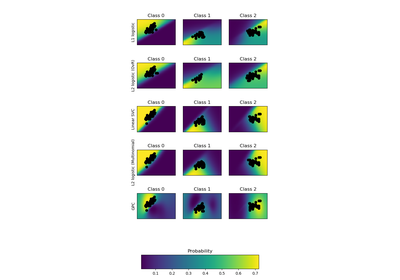Plot classification probability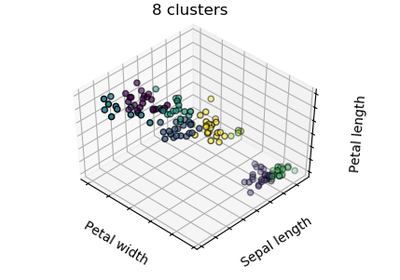K-means Clustering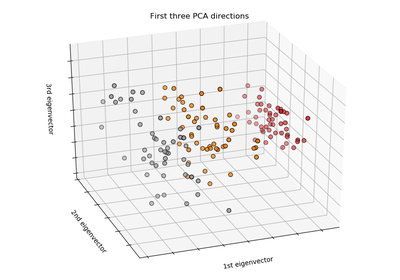The Iris Dataset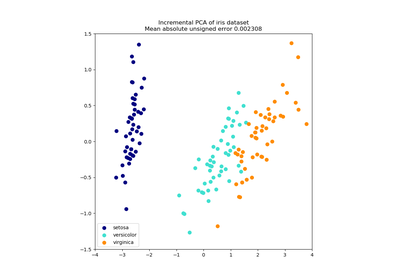Incremental PCA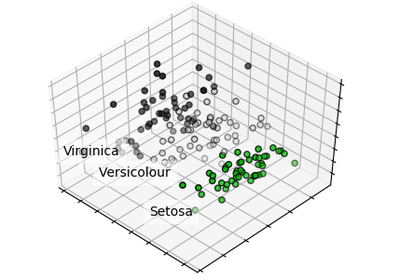PCA example with Iris Data-set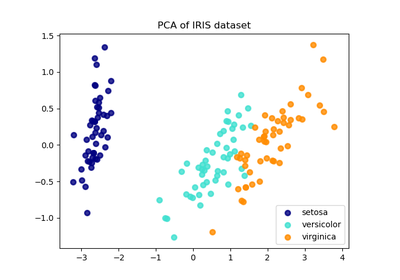Comparison of LDA and PCA 2D projection of Iris dataset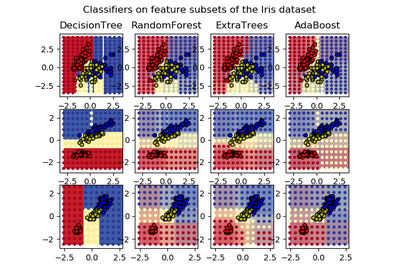Plot the decision surfaces of ensembles of trees on the iris dataset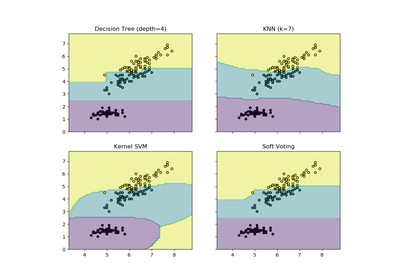Plot the decision boundaries of a VotingClassifier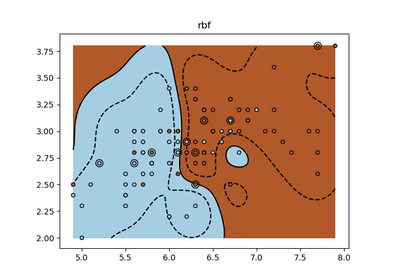SVM Exercise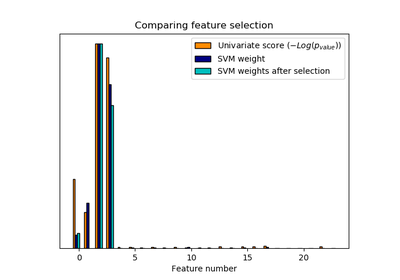Univariate Feature Selection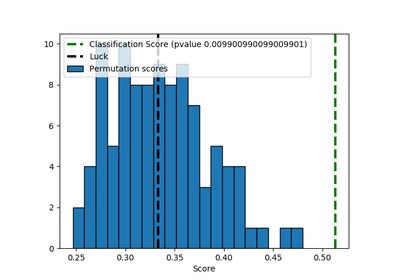Test with permutations the significance of a classification score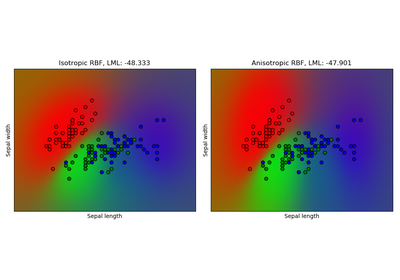Gaussian process classification (GPC) on iris dataset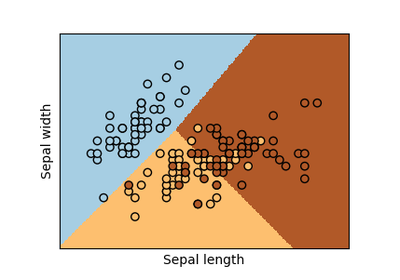Logistic Regression 3-class Classifier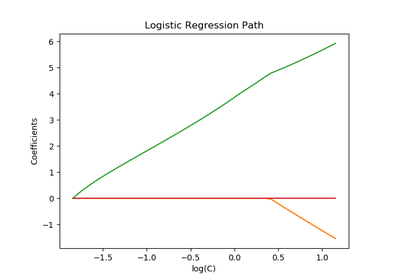Path with L1- Logistic Regression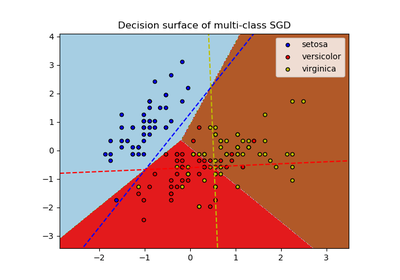Plot multi-class SGD on the iris dataset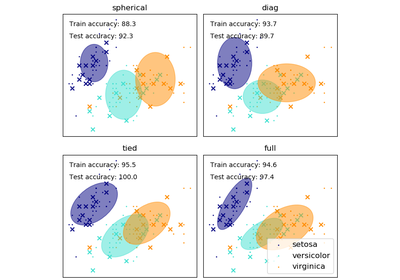GMM covariances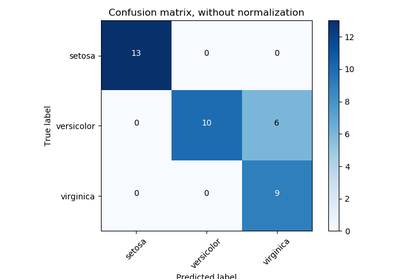Confusion matrix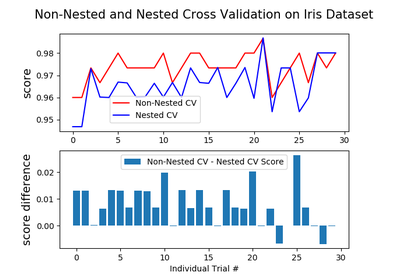Nested versus non-nested cross-validation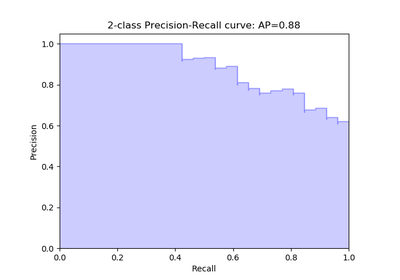Precision-Recall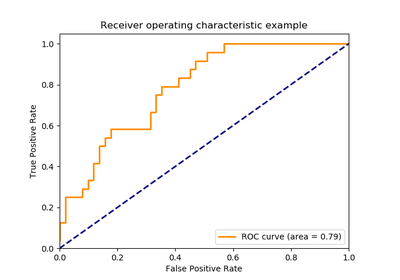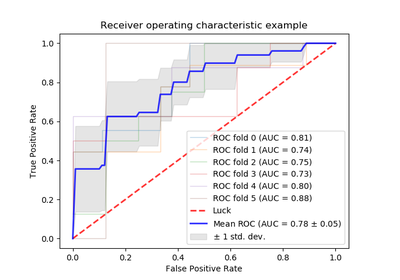Receiver Operating Characteristic (ROC) with cross validation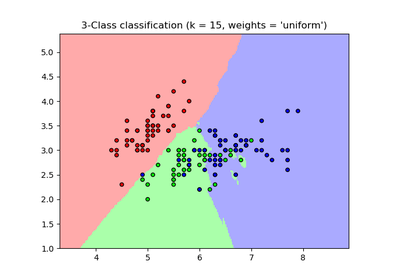Nearest Neighbors Classification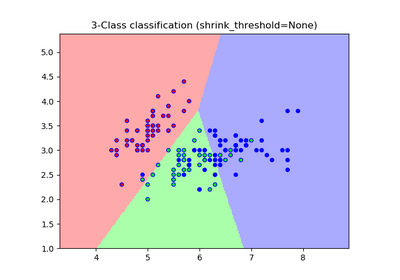Nearest Centroid Classification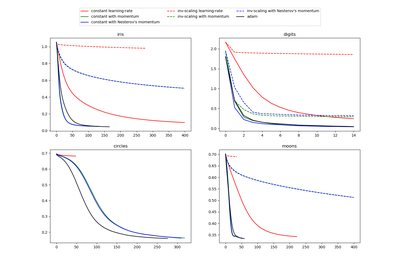Compare Stochastic learning strategies for MLPClassifier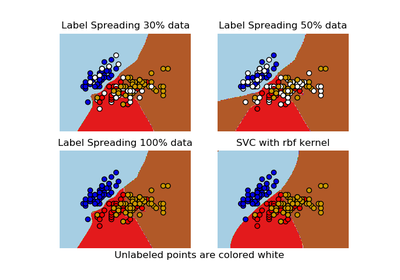Decision boundary of label propagation versus SVM on the Iris dataset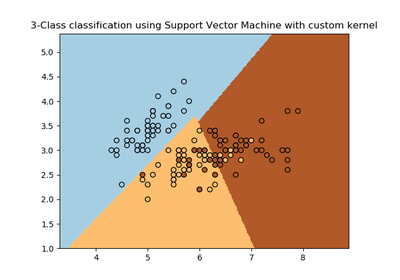SVM with custom kernel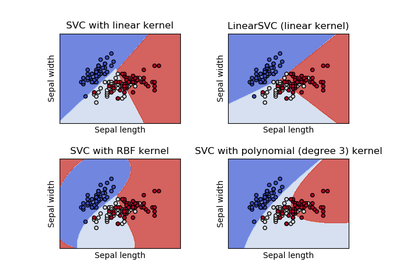Plot different SVM classifiers in the iris dataset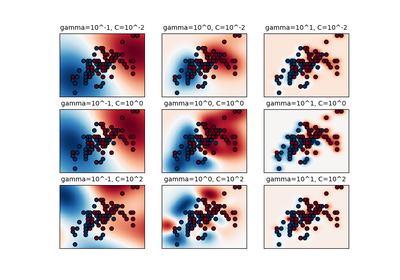RBF SVM parameters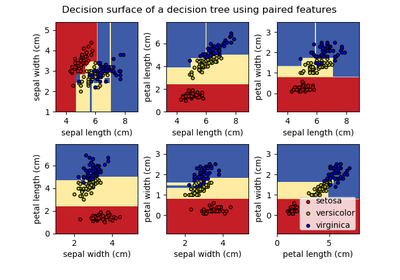Plot the decision surface of a decision tree on the iris datasetUnderstanding the decision tree structure